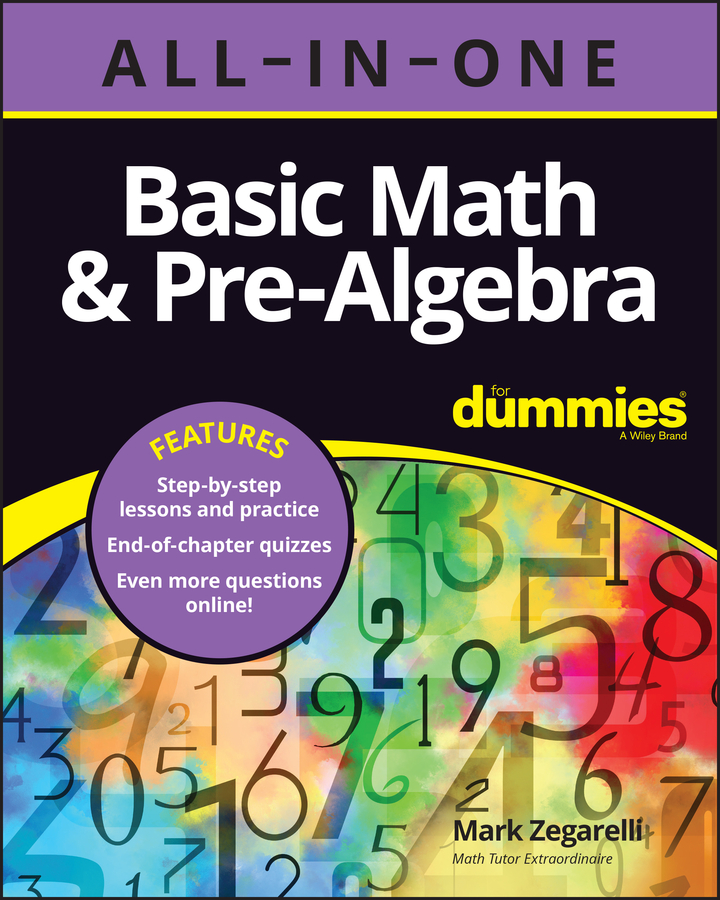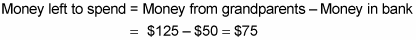##### Basic Math & Pre-Algebra All-in-One For Dummies (+ Chapter Quizzes Online)The word of in a word problem usually means multiplication. This idea is also true in word problems involving decimals and percents. The method for solving these two types of problems is similar, as you’ll see below.

You can easily solve word problems involving percents by changing the percents into decimals. For example, here are a few common percents and their decimal equivalents:

25% = 0.25 50% = 0.5 75% = 0.75 99% = 0.99

One common type of problem gives you a starting amount — and a bunch of other information — and then asks you to figure out how much you end up with. Here’s an example:

Maria’s grandparents gave her \$125 for her birthday. She put 40% of the money in the bank, spent 35% of what was left on a pair of shoes, and then spent the rest on a dress. How much did the dress cost?

Start at the beginning, forming a word equation to find out how much money Maria put in the bank:

Money in bank = 40% of \$125

To solve this word equation, change the percent to a decimal and the word of to a multiplication sign; then multiply:

Money in bank = 0.4 \$125 = \$50

Pay special attention to whether you’re calculating how much of something was used up or how much of something is left over. If you need to work with the portion that remains, you may have to subtract the amount used from the amount you started with.

Because Maria started with \$125, she had \$75 left to spend:The problem then says that she spent 35% of this amount on a pair of shoes. Again, change the percent to a decimal and the word of to a multiplication sign:

Shoes = 35% of \$75 = 0.35 \$75 = \$26.25

She spent the rest of the money on a dress, so

Dress = \$75 – \$26.25 = \$48.75

Therefore, Maria spent \$48.75 on the dress.